CLAT  >  Practice Test: Logical Reasoning - 4

# Practice Test: Logical Reasoning - 4

Test Description

## 40 Questions MCQ Test Logical Reasoning for CLAT | Practice Test: Logical Reasoning - 4

Practice Test: Logical Reasoning - 4 for CLAT 2022 is part of Logical Reasoning for CLAT preparation. The Practice Test: Logical Reasoning - 4 questions and answers have been prepared according to the CLAT exam syllabus.The Practice Test: Logical Reasoning - 4 MCQs are made for CLAT 2022 Exam. Find important definitions, questions, notes, meanings, examples, exercises, MCQs and online tests for Practice Test: Logical Reasoning - 4 below.
Solutions of Practice Test: Logical Reasoning - 4 questions in English are available as part of our Logical Reasoning for CLAT for CLAT & Practice Test: Logical Reasoning - 4 solutions in Hindi for Logical Reasoning for CLAT course. Download more important topics, notes, lectures and mock test series for CLAT Exam by signing up for free. Attempt Practice Test: Logical Reasoning - 4 | 40 questions in 30 minutes | Mock test for CLAT preparation | Free important questions MCQ to study Logical Reasoning for CLAT for CLAT Exam | Download free PDF with solutions
 1 Crore+ students have signed up on EduRev. Have you?
Practice Test: Logical Reasoning - 4 - Question 1

### Each group of question in this section is based on a set of conditions. Choose the response that most accurately and completely answer each question. A panel reviews six contract bids- H, J, K, R, S, and T. No two bids have the same cost. Exactly one of the bids is accepted. The following conditions must hold: 1) The accepted bid is either K or R and is either the second or the third lowest in cost 2) H is lower in cost than each of J and K 3) If J is the fourth lowest in cost, than J is higher in cost than each of S and T 4) If J is not the fourth lowest in cost, than J is higher in cost than each of S and T 5) Either R or S is the fifth lowest in cost. Q. Which one of the following could be an accurate list of the bids in order from lowest to highest in cost?

Detailed Solution for Practice Test: Logical Reasoning - 4 - Question 1

Lowest Bid Possibility
1] S/T/H
2] S/T/H/K
3] S/T/H/K
4] S/T/H
5] R
Highest Bid 6] J

Practice Test: Logical Reasoning - 4 - Question 2

### Each group of question in this section is based on a set of conditions. Choose the response that most accurately and completely answer each question. A panel reviews six contract bids- H, J, K, R, S, and T. No two bids have the same cost. Exactly one of the bids is accepted. The following conditions must hold: 1) The accepted bid is either K or R and is either the second or the third lowest in cost 2) H is lower in cost than each of J and K 3) If J is the fourth lowest in cost, than J is higher in cost than each of S and T 4) If J is not the fourth lowest in cost, than J is higher in cost than each of S and T 5) Either R or S is the fifth lowest in cost. Q.  Which one of the following bids CANNOT be the fourth lowest in cost?

Practice Test: Logical Reasoning - 4 - Question 3

### Read carefully the question and select the most appropriate answer.Q.​​ Plato said that ‘government is the best government which governs least.’ What does this statement contradict from among the following statements?

Detailed Solution for Practice Test: Logical Reasoning - 4 - Question 3

The correct option is B.
An able government legislates on every aspect of a citizen's life contradicts the given statement as the statement states that ‘government is the best government which governs least.’ but this statement talks about strictness by the government.

Practice Test: Logical Reasoning - 4 - Question 4

In each of the following questions, a number series is given with one term missing. Choose the correct alternative that will continue the same pattern and fill in the black spaces.

Q.

95,115.5, 138, (___), 189

Detailed Solution for Practice Test: Logical Reasoning - 4 - Question 4

EXP: 162.5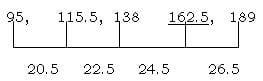In difference +2 is added so Next is 22.5 +2= 24.5, 24.5+2=26.5

Practice Test: Logical Reasoning - 4 - Question 5

Read the given information carefully and answer the questions based on them:

Six members of a family A, B, C, D, E and F are Psychologist, Manager, Advocate, Jeweller, Doctor and Engineer but not in the same order

1. Doctor is the grandfather of F who is a Psychologist.
2. Manager D is married to A.
3. C, who is a Jeweller, is married to Advocate.
4. B is the mother of F and E.
5. There are two married couples in the family.

Q.
How many male members are there in the family?

Detailed Solution for Practice Test: Logical Reasoning - 4 - Question 5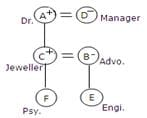Practice Test: Logical Reasoning - 4 - Question 6

Read carefully the question and select the most appropriate answer.

Q.​
A straight line is defined as the shortest distance between any two points consisting of infinite number of dimensionless point. If this definition is acceptable, which of the following logically follows from the given definition?

Practice Test: Logical Reasoning - 4 - Question 7

Read carefully the question and select the most appropriate answer.

Q.​
Four probable statements are given (not necessarily related) which are to be arranged in the ascending order of probability. From among the alternatives, identify the correct arrangement.

A) The sun will rise tomorrow. (A)

B) This year there will be copious rainfall. (B)

C) Tomorrow it will rain. (C)

D) Every smoker will have attack before he or she turns 40 years. (D)

Detailed Solution for Practice Test: Logical Reasoning - 4 - Question 7

The correct arrangement is C as according  to probability the statements are correctly arranged.

Practice Test: Logical Reasoning - 4 - Question 8

If it is true that All men are honest and All politicians are men, for which among the following is it impossible to follow from the given premises?

Detailed Solution for Practice Test: Logical Reasoning - 4 - Question 8

The correct option is A.

Other than A all the conclusions are possible from the given statements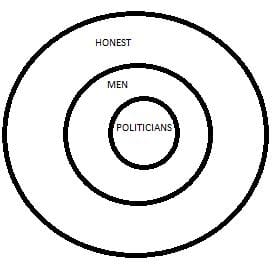Practice Test: Logical Reasoning - 4 - Question 9

In each question below are given two statements followed by two conclusions numbered I and II. You have to take the given two statements to be true even if they seem to be at variance from commonly known facts. Read the conclusions and then decide which of the given conclusions logically follows from the two given statements, disregarding commonly known facts.

Q.
Statement: Some pastries are toffees.

All toffees are chocolates.

Conclusions:

I. Some chocolates are toffees.

II. Some toffees are not pastries.

Detailed Solution for Practice Test: Logical Reasoning - 4 - Question 9

The correct option is D.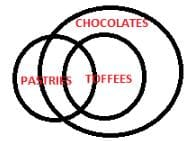Practice Test: Logical Reasoning - 4 - Question 10

Each group of question in this section is based on a set of conditions. Choose the response that most accurately and completely answer each question.

A panel reviews six contract bids- H, J, K, R, S, and T. No two bids have the same cost. Exactly one of the bids is accepted. The following conditions must hold:

1) The accepted bid is either K or R and is either the second or the third lowest in cost

2) H is lower in cost than each of J and K

3) If J is the fourth lowest in cost, than J is higher in cost than each of S and T

4) If J is not the fourth lowest in cost, than J is higher in cost than each of S and T

5) Either R or S is the fifth lowest in cost.

Q.
Which one of the following bids CANNOT be the second lowest in cost?

Detailed Solution for Practice Test: Logical Reasoning - 4 - Question 10

The correct option is A.
From the given statements, following arrangements are possible
J R S K T H
J S R K T H
J R S K T H
J S K R T H
J R T S  K H
J S T K R H

Practice Test: Logical Reasoning - 4 - Question 11

Read carefully the question and select the most appropriate answer.

Q.​
Consider the statement, ‘in a remote stellar system there may be a planet with some form of life or other.’ How do you evaluate this statement?

Detailed Solution for Practice Test: Logical Reasoning - 4 - Question 11

The correct option is B.
The statement is unreasonable and illogical.

Practice Test: Logical Reasoning - 4 - Question 12

Read carefully the question and select the most appropriate answer.

Q.
While pursing research on thermodynamics, Carnot proposed ‘ideal heat engines’ which cannot be realised, with the help of which the second law of thermodynamics was formulated. What does the reference to ‘ideal heat engines’ suggest?

Practice Test: Logical Reasoning - 4 - Question 13

Read carefully the question and select the most appropriate answer.

Q.
​​Four judgments are given, out of which one is neither a crime nor a sin. Identify the same.

Practice Test: Logical Reasoning - 4 - Question 14

Read carefully the question and select the most appropriate answer.

Q.
If it is true that no men are cannibals and cannibals do not exist, then which of the following alternatives must be accepted as true conclusion.

Detailed Solution for Practice Test: Logical Reasoning - 4 - Question 14

EXP: No true conclusion is possible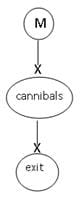Practice Test: Logical Reasoning - 4 - Question 15

In each question below are given two statements followed by two conclusions numbered I and II. You have to take the given two statements to be true even if they seem to be at variance from commonly known facts. Read the conclusions and then decide which of the given conclusions logically follows from the two given statements, disregarding commonly known facts.

Q.
Statement: Some calendars are sticks.

No stick is flower.

Conclusions:

I. Some calendars are flowers

II. No calendar is flower.

Detailed Solution for Practice Test: Logical Reasoning - 4 - Question 15

The correct option is D.
Since one premise is particular, the conclusion must be particular. So, II cannot follow.
Since one premise is negative, the conclusion must be negative. So, I cannot follow.

Practice Test: Logical Reasoning - 4 - Question 16

Mark the correct inference drawn in each case.

Q.​
Statement: Many smokes suffer from cancer.Inferences:

Practice Test: Logical Reasoning - 4 - Question 17

In a queue of 20 boys, D is fourteenth from the front and F is ninth from the bottom. How many boys are there between D and F?

Detailed Solution for Practice Test: Logical Reasoning - 4 - Question 17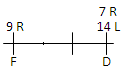Question is based on minimum no. of students :-

Minimum No. of Students = 1

D’s rank from Right (R) = Total no. of Boys + 1 – D’s Rank from Left (L)

20 + 1 – 14

= 7 R.

∴7 R – 9 R = 1 student

Practice Test: Logical Reasoning - 4 - Question 18

Read carefully the question and select the most appropriate answer.

Q.​​
Four statements are given. Arrange them in the descending order of probability using the alternatives provided below the statements.

1. It is probable that when three coins are tossed simultaneously all of them show heads. (A)

2. It is possible that when the same die with 6 faces is cast twice, it shows 2. (B)

3. When three packs of playing cards are mixed thoroughly, the card pulled out first may be spade king. (C)

4. When the first card pulled out from a well, mixed three packs of playing cards is spade king, the next card pulled out also may be spade king.

Practice Test: Logical Reasoning - 4 - Question 19

Mark the correct inference drawn in each case.

Q.​
Statement: Examinations are going on in the Law Faculty. The Law Faculty is closed on Sunday.Inferences:

Practice Test: Logical Reasoning - 4 - Question 20

Read carefully the question and select the most appropriate answer.

Q.
Followers of a certain school of philosophy argued that it is impossible to conclusively verify a statement, it is meaningless. If so, against this background, choose the most appropriate answer from among the alternatives given below to determine the status of the statement – consciousness is the manifestation of divine will?

Practice Test: Logical Reasoning - 4 - Question 21

Mark the correct inference drawn in each case.

Q.
Statement: Most of the students of Navodaya Vidyalaya are of outstanding merit.Inferences:

Practice Test: Logical Reasoning - 4 - Question 22

Read the given information carefully and answer the questions based on them:

Six members of a family A, B, C, D, E and F are Psychologist, Manager, Advocate, Jeweller, Doctor and Engineer but not in the same order

1. Doctor is the grandfather of F who is a Psychologist.
2. Manager D is married to A.
3. C, who is a Jeweller, is married to Advocate.
4. B is the mother of F and E.
5. There are two married couples in the family.

Q.
What is the profession of E?

Detailed Solution for Practice Test: Logical Reasoning - 4 - Question 22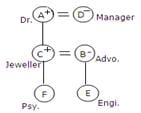Practice Test: Logical Reasoning - 4 - Question 23

In each of the following questions, a number series is given with one term missing. Choose the correct alternative that will continue the same pattern and fill in the black spaces.

Q. 1, 3, 4, 8, 15, 27, (___)

Detailed Solution for Practice Test: Logical Reasoning - 4 - Question 23

EXP: 50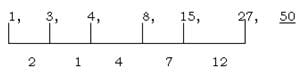We have calculated difference but no conclusion arrived. We see

1 = 1

3 = 3

4 = 3+1

8 = 4+3+1

15 = 8+4+3

27 =15+8+4

50 = 27+15+8

By Adding last three numbers, we get the value of next no.

Practice Test: Logical Reasoning - 4 - Question 24

If Reena says, “Anjali's father Raman is the only son of my father-in-law Ramanand”, then how is Piyu, who is the sister of Anjali, related to Ramanand ?​

Detailed Solution for Practice Test: Logical Reasoning - 4 - Question 24

Only son of Reena's father-in-law Ramanand is Reena's husband. So, Raman is Reena's husband and Anjali & Piyu are his daughter's, Thus, Piyu is Grand-daughter of Ramanand.

Practice Test: Logical Reasoning - 4 - Question 25

Read carefully the question and select the most appropriate answer.

Q.​
If it is true that neither Rama is a brother of Krishna, nor is he a lecturer, then which one of the following statements is true?

Detailed Solution for Practice Test: Logical Reasoning - 4 - Question 25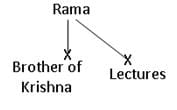Practice Test: Logical Reasoning - 4 - Question 26

If is possible to make a meaningful word with the second the fourth, the sixth and the ninth letters of RECTIFICATION, what will be the third letter of the word? If no such word is possible mark X as your answer and if more than one word is possible mark M as your answer.

Detailed Solution for Practice Test: Logical Reasoning - 4 - Question 26

EXP: M  RECTIFICATION          2, 4, 6, 9th Letter

Using these Four words E, T, F, A Two words formed i.e Feat, Fate. More than one word is formed so

Practice Test: Logical Reasoning - 4 - Question 27

Read carefully the question and select the most appropriate answer.

Q.
Some philosophers argue that what does not exist is not knowable. Which of the following alternatives is implied by this claim?

Detailed Solution for Practice Test: Logical Reasoning - 4 - Question 27

The correct option is A
Different scientists and discoverers have different points of view. The things that still don't exist are knowable. Example - life on mars.

Practice Test: Logical Reasoning - 4 - Question 28

There is a certain relation between two given words on one side of::and one word is given on another side of::while another word is to be found from the given alternatives, having the same relation with this word as the words of the given pair bear. Choose the best alternative.

Q.
Watch: Second :: Calendar : _____

Detailed Solution for Practice Test: Logical Reasoning - 4 - Question 28

EXP: Date

1st is used to view 2nd

Practice Test: Logical Reasoning - 4 - Question 29

Read carefully the question and select the most appropriate answer.

Q.
It is argued that all democratic systems of government breed corruption, but at the same time protect human rights. If so, which of the following alternatives is evidently false?

Detailed Solution for Practice Test: Logical Reasoning - 4 - Question 29

The correct option is A.
It is the best suitable option.Because it is given in question that democracy is corrupt but it still protects human rights but the C option says that democracy is corrupt and does not protect human rights which is false. And option D is not correct at all. Option B gives priority to corruption.

Practice Test: Logical Reasoning - 4 - Question 30

Identify the conclusion which follows from the premises given below:Premises:If Rajiv has committed the crime, he will be arrested.If he is arrested, then he will be prosecuted.If he is prosecuted, then he will be imprisoned.Therefore,

Practice Test: Logical Reasoning - 4 - Question 31

Read carefully the question and select the most appropriate answer.

Q.​
​In the context of crime committed, four evidences are considered which are mentioned below. Arrange them in the increasing order of their weightage.

1. Computer-generated image based on the description given by a neighbour.

2. A piece of paper at the site of crime containing an address of suspect.

3. Matching of finger prints.

4. Tracing the call made by the suspect to the victim

Practice Test: Logical Reasoning - 4 - Question 32

Read carefully the question and select the most appropriate answer.

Q.
​​Four judgments are given out of which one judgment regards the action as both crime and sin. Identify the same.

Practice Test: Logical Reasoning - 4 - Question 33

In each question below are given two statements followed by two conclusions numbered I and II. You have to take the given two statements to be true even if they seem to be at variance from commonly known facts. Read the conclusions and then decide which of the given conclusions logically follows from the two given statements, disregarding commonly known facts.

Q.
Statement: Some boys are men.

No men is black.

Conclusions:

I. Some boys are not black.

II. Some men are boys.

Detailed Solution for Practice Test: Logical Reasoning - 4 - Question 33

The correct option is A.
Since one premise is negative, the conclusion must be negative. So. II does not follow
and only I follow.

Practice Test: Logical Reasoning - 4 - Question 34

Assume that there are three statements followed by several conclusions. You have to take the given statements to be true, even if they seem to be at variance from commonly-known facts and then decide which of the given conclusion/s logically follows.
Statements :
I. All Pens are Tables
II. Some tables are rulers.
III. Some rulers are chalk.

Conclusion :
I. Some tables are chalk.
II. Some pens are chalk.
III. Some rulers are pens.
IV. Some chalk are rulers

Detailed Solution for Practice Test: Logical Reasoning - 4 - Question 34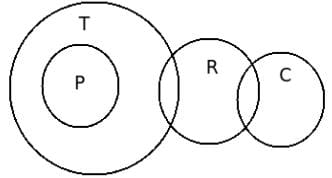This diagram tells us that only one condition follows i.e. some chalk are rulers.

Practice Test: Logical Reasoning - 4 - Question 35

In a certain code language BLUNDER is written as LBNURED. Then how will QUICKER be written in that code?

Detailed Solution for Practice Test: Logical Reasoning - 4 - Question 35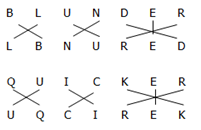Practice Test: Logical Reasoning - 4 - Question 36

There is a certain relation between two given words on one side of::and one word is given on another side of::while another word is to be found from the given alternatives, having the same relation with this word as the words of the given pair bear. Choose the best alternative.

Q.
Student: Teacher :: Player:_____

Detailed Solution for Practice Test: Logical Reasoning - 4 - Question 36

EXP: Coach

2nd is guide of 1st

Practice Test: Logical Reasoning - 4 - Question 37

Read the questions carefully and mark which one is right.

Q.
Plato said ‘that government is the best government which governs least’. What does this statement contradict from among the following statements?

Detailed Solution for Practice Test: Logical Reasoning - 4 - Question 37

The correct option is B.
The question states that the best government is one that governs the least that means it talks about the freedom given by the government to the citizens but on the other hand this option talks about the restrictions by the government.

Practice Test: Logical Reasoning - 4 - Question 38

Read carefully the question and select the most appropriate answer.

Q.
Identify the statement which is false if it is true that no beings other than men are intelligent.

Detailed Solution for Practice Test: Logical Reasoning - 4 - Question 38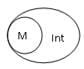Practice Test: Logical Reasoning - 4 - Question 39

Read the questions carefully and mark which one is right.

Q.
While pursuing research on thermodynamics, Carnot proposed ‘ideal heat engines’, which cannot be realized, with the help of which the second law of thermodynamics was formulated.

What does the reference to ‘ideal’ heat engines suggest?

Practice Test: Logical Reasoning - 4 - Question 40

Jenny walked 2.5 km’s towards North and turned towards West. After covering 2 km’s he turned to South and walked 1.5 km’s. He then turned to East and covered 2 km’s. To which direction Jenny is standing from the original point.

Detailed Solution for Practice Test: Logical Reasoning - 4 - Question 40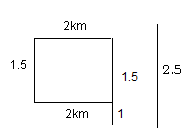North

## Logical Reasoning for CLAT

19 videos|24 docs|67 tests
 Use Code STAYHOME200 and get INR 200 additional OFF Use Coupon Code
Information about Practice Test: Logical Reasoning - 4 Page
In this test you can find the Exam questions for Practice Test: Logical Reasoning - 4 solved & explained in the simplest way possible. Besides giving Questions and answers for Practice Test: Logical Reasoning - 4, EduRev gives you an ample number of Online tests for practice

## Logical Reasoning for CLAT

19 videos|24 docs|67 tests